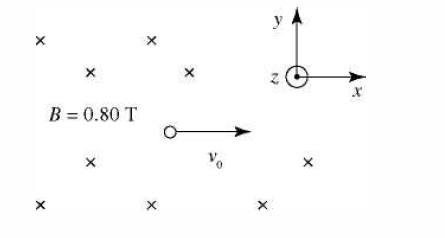# Electron passing through a magnetic and an electric field

## Homework Statement

An electron with velocity ##\vec{v}_0=8.7*10^4(m/s)## (in the î direction) passing through an area with a uniform magnetic field ##\vec{B}=0.80 T## (in the negative k̂ direction). There's also a uniform electric field in this area.

What is the magnitude and direction of this electric field if the electron travels through this area without being deflected? [see picture below]## Homework Equations

The Lorentz force ##\vec{F}=q(\vec{E}+\vec{v}×\vec{B})##
Right Hand Rule

## The Attempt at a Solution

[/B]
The speed is low in comparison to that of the speed of light and the Lorentz factor ##γ=1.000000042## so we won't deal with special relativity in this problem.

So first let's use the RHR to decide the direction of the magnetic force on the electron. The crossproduct ##(\vec{v}×\vec{B})## multiplied by the negative scalar ##q## (since we're dealing with an electron) gives the magnetic force to point downward. The electric field that we seek has to point upwards and be equal to the magnetic force to make the electron stay fixed in its y-position.

The two parts of the Lorentz force has to be equal.

##\vec{F}_B=\vec{F}_E## and since we're dealing with uniform fields and the magnetic field and the velocity are perpendicular to eachother the ##sin(θ)## is just ##1##.

We also know the direction of all the fields (the direction ##E##-field follows from definition and the wanted result in this problem) so we can skip the vector signs and simply have:
##qvB=qE## and ##E=vB##.

So the electric field has to point upward in the picture and have a magnitude of:
##E=(8.7*10^4 (m/s))(0.80 T)=6.09*10^3 (N/C)##

Did I get it right and is my reasoning on point?

TSny
Homework Helper
Gold Member
Everything looks good to me except your result for multiplying (8.7 x 104)(0.80). Since you have coordinate axes given, it might be a little better to express the direction of the answer as "in the +y direction" or "in the ##\hat{j}## direction".

•